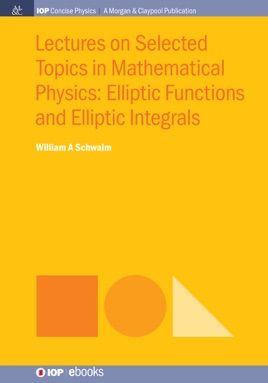• USD 28.99

## Descripción de editorial

This volume is a basic introduction to certain aspects of elliptic functions and elliptic integrals. Primarily, the elliptic functions stand out as closed solutions to a class of physical and geometrical problems giving rise to nonlinear differential equations. While these nonlinear equations may not be the types of greatest interest currently, the fact that they are solvable exactly in terms of functions about which much is known makes up for this. The elliptic functions of Jacobi, or equivalently the Weierstrass elliptic functions, inhabit the literature on current problems in condensed matter and statistical physics, on solitons and conformal representations, and all sorts of famous problems in classical mechanics.

The lectures on elliptic functions have evolved as part of the first semester of a course on theoretical and mathematical methods given to first and second year graduate students in physics and chemistry at the University of North Dakota. They are for graduate students or for researchers who want an elementary introduction to the subject that nevertheless leaves them with enough of the details to address real problems. The style is supposed to be informal. The intention is to introduce the subject as a moderate extension of ordinary trigonometry in which the reference circle is replaced by an ellipse. This entre depends upon fewer tools and has seemed less intimidating that other typical introductions to the subject that depend on some knowledge of complex variables. The first three lectures assume only calculus, including the chain rule and elementary knowledge of differential equations. In the later lectures, the complex analytic properties are introduced naturally so that a more complete study becomes possible.